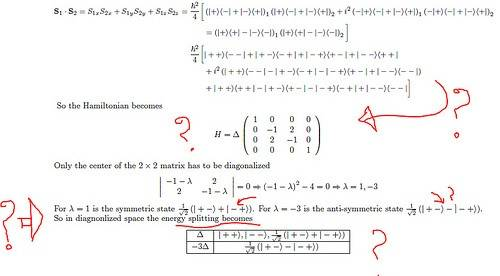# Need explanation of the solutions of Sakurai Quantum Mechanics Problem 29 of chap 5

## Homework Statement

I have attached a link to the solution set of some questions from sakurai. But I dont understand clearly the solution of question number 5.29.

## Homework Equations

how did they found the 4x4 matrix from the spin product, how did they get the complete spectrum etc. etc. it would very helpful, if someone would explain it. It is so much urgent.

## The Attempt at a Solution

I need a explained solution. It is urgent

Last edited by a moderator:

berkeman
Mentor

## Homework Statement

I have attached a link to the solution set of some questions from sakurai. But I dont understand clearly the solution of question number 5.29.

## Homework Equations

how did they found the 4x4 matrix from the spin product, how did they get the complete spectrum etc. etc. it would very helpful, if someone would explain it. It is so much urgent.

## The Attempt at a Solution

I need a explained solution. It is urgent

Link does not appear to be working...

Last edited by a moderator:

Link does not appear to be working...

http://www.people.virginia.edu/~rdb2k/homework/phys752/quantum.pdf" [Broken]

Reminding: Question no. 5.29.
Questions: how did they found the 4x4 matrix from the spin product, how did they get the complete spectrum etc. etc. it would very helpful, if someone would explain it. It is so much urgent.

Last edited by a moderator:
Need explanation of this QM problem

## Homework Statement

I have attached a link to the solution set of some questions from sakurai. But I dont understand clearly the solution of question number 5.29.
http://www.people.virginia.edu/~rdb2k/homework/phys752/quantum.pdf" [Broken]

## Homework Equations

how did they found the 4x4 matrix from the spin product, why only middle 2x2 matrix of this 4x4 matrix need to diagonalize, how did they get the complete spectrum i.e. |++>, |-->. etc etc after calculating eigenvalues. it would very helpful, if someone would explain it. It is so much urgent.

## The Attempt at a Solution

Last edited by a moderator:

Well I think these may help u better understand...
http://en.wikipedia.org/wiki/Hamiltonian_matrix
http://en.wikipedia.org/wiki/Hamiltonian_(quantum_mechanics [Broken])
http://www.nivi.com/papers/hamiltonian.pdf [Broken]
http://lheawww.gsfc.nasa.gov/users/audley/msctest/node18.html [Broken]

Only the 2x2 middle matrix needs to be diagonalised as the other two values on the boundary are already 1 on the diagonal and the rest are 0's.. for A diagonal matrix only the diagonal elements can have a value (including 0)... other elements need to be 0.
This is the requirement of a diagonal matrix.. It is good to have a diagonal matrix as it helps to simplify the result determination process. And it is valid as any manipulation of the elements of the matrix does not affect the determinant or value of the entire matrix.

Last edited by a moderator:

how did they found the 4x4 matrix from the spin product, why only middle 2x2 matrix of this 4x4 matrix need to diagonalize, how did they get the complete spectrum i.e. |++>, |-->. etc etc after calculating eigenvalues.

Please look into this to understand solving matrices through eigen value manipulation.
http://en.wikipedia.org/wiki/Eigenvalue_algorithm

and look into this for definition of Diagonal matrix..
http://en.wikipedia.org/wiki/Diagonal_matrix

If you have the time .. do glance through this link... It will keep u more informed on the nature and types of matrices...
http://en.wikipedia.org/wiki/Matrix_(mathematics [Broken])

Last edited by a moderator:
berkeman
Mentor

Actually, my question is I don't understand the portion indicated in the picture attached. Please see the picture. How they got 4x4 matrix from the previous line, and how they got symmetric and antisymmetric states for those eigenvalues, 1 and -3 respectively and also, how the got the total enery splitting. I am quite weak in these matter. It would be better for me, if you could explain these.

Picture:Capture by mshvn, on Flickr

for the diagonal matrix they have found the eigen values considering the determinant of the matrix as 0. Eigen values are the ones that when inserted into the matrix result the determinant of the matrix to be 0.
solving the determinant we get as in the picture:: ((-1-L)x(-1-L))-(2*2) = 0;
~= (-1-L)^2 - 4 = 0
hence (-1-L)^2 = 4
Taking sq. root on both sides we get two possible equations;
-1-L = 2 or 1+L=2
hence we obtain 2 possible values of L to be ; L=-3 // L=1

for the diagonal matrix they have found the eigen values considering the determinant of the matrix as 0. Eigen values are the ones that when inserted into the matrix result the determinant of the matrix to be 0.
solving the determinant we get as in the picture:: ((-1-L)x(-1-L))-(2*2) = 0;
~= (-1-L)^2 - 4 = 0
hence (-1-L)^2 = 4
Taking sq. root on both sides we get two possible equations;
-1-L = 2 or 1+L=2
hence we obtain 2 possible values of L to be ; L=-3 // L=1

I know how to solve eigenvalues. My question is not that. I actually dont understand how they get the 4x4 matrix from the previous line of spin product expression? I mean, they directly wrote the Hamiltonian as 4x4 matrix from the previous line. I dont know how they did that from that spin product?

fzero
Homework Helper
Gold Member

There is a basis for the space of two particle states which is $$\{ |++\rangle, |+-\rangle,|-+\rangle,|--\rangle \}$$. We can choose an index $$i$$ and label these states as $$| i \rangle$$ for $$i = 1,2,3,4$$. Then the Hamiltonian can be written

$$\hat{H} = \sum_{ij} H_{ij} | i \rangle \langle j |.$$

The coefficients $$H_{ij}$$ are the elements of the matrix corresponding to $$\hat{H}$$.